# GSEB Solutions Class 12 Statistics Part 1 Chapter 1 Index Number Ex 1.4

Gujarat Board Statistics Class 12 GSEB Solutions Part 1 Chapter 1 Index Number Ex 1.4 Textbook Exercise Questions and Answers.

## Gujarat Board Textbook Solutions Class 12 Statistics Part 1 Chapter 1 Index Number Ex 1.4

Question 1.
The following data are obtained from the family budget inquiry of middle class people. State the change in the cost of living in the year 2015 with respect to the year 2013 by finding the index number. If the average monthly disposable income of a family in the year 2013 is ₹ 15000, then obtain the estimate of the necessary average monthly disposable income in the year 2015.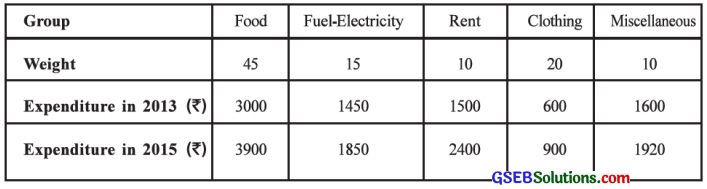Here, p0 = Expenditure in 2013 and P1 = Expenditure in 2015.
The table for calculation is prepared as follows: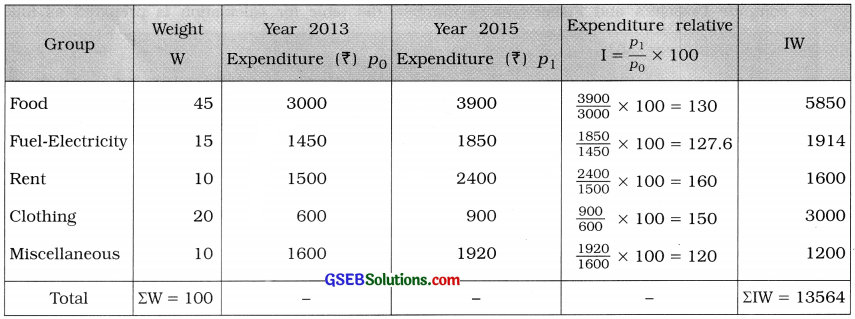Cost of living index number:
I = $$\frac{\Sigma \mathrm{IW}}{\Sigma \mathrm{W}}$$ = $$\frac{13564}{100}$$ = 135.64
The cost of living in the year 2015 has been increased (135.64 – 100 =) 35.64 %
The necessary average monthly disposable income in the year 2015
= $$\frac{(\text { The average monthly income in 2013) } \times(\text { The cost of living index number in 2015) }}{100}$$
= $$\frac{15000 \times 135.64}{100}$$
= $$\frac{2034600}{100}$$
= ₹ 20346Question 2.
Find the index number for the year 2014 by the method of family budget from the following data about prices and consumption of food items and interpret it: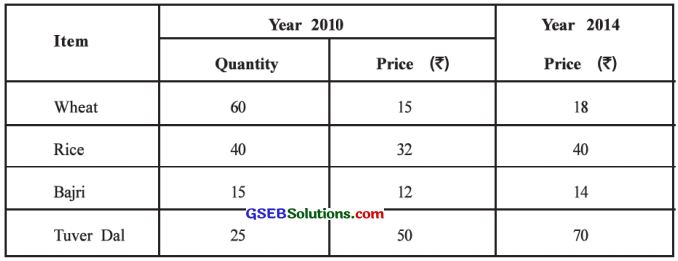Here, p0 = Price in 2010, q0 = Quantity in 2010 and p1 = Price in 2014.
The table for calculation is prepared as follows: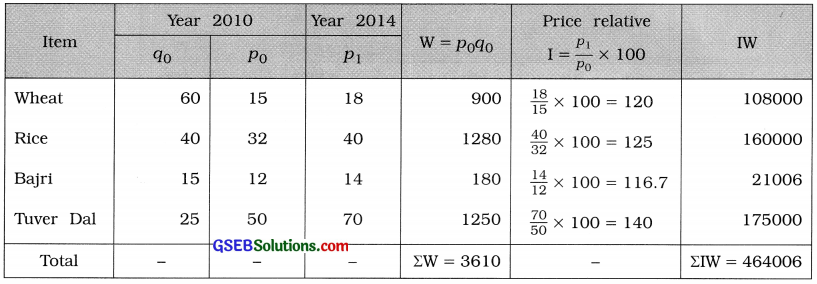Index number of 2014 by family budget method:
I = $$\frac{\Sigma \mathrm{IW}}{\Sigma \mathrm{W}}$$
= $$\frac{464006}{3610}$$
= 128.53

Interpretation: Index number of 2014 is 128.53. So there is (128.53 – 100 =) 28.53 % increase in the prices of food items for the year 2014.Question 3.
Compute the cost of living index number by the method of total expenditure from the following data: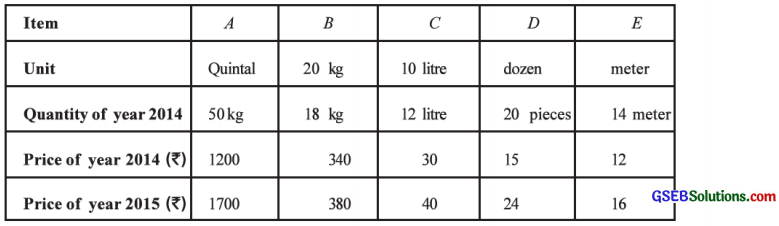Here, p0 = Price in 2014, q0 = Quantity in 2014 and p1 = Price in 2015.
After making the units of price and quantity same, we will compute index number.

Explanation:
Item A: The unit of price is quintal and the unit of quantity is kg.
∴ In 2014. the price per kg = $$\frac{1200}{100}$$ = ₹ 12
In 2015, the price per kg = $$\frac{1700}{100}$$ = ₹ 17

Item B : The unit of price is 20 kg and the unit of quantity is kg.
∴ In 2014. the price per kg = $$\frac{340}{20}$$ = ₹ 17
In 2015, the price per kg = $$\frac{380}{20}$$ = ₹ 19

Item C: The unit of price is 10 litre and the unit of quantity is litre.
∴ In 2014, the price per litre = $$\frac{30}{10}$$ = ₹ 3
In 2015, the price per litre = $$\frac{40}{10}$$ = ₹ 4

Item D : The unit of price is dozen and the unit of quantity is a piece.
∴ In 2014, the price per piece = $$\frac{15}{12}$$ = ₹ 1.25
In 2015, the price per piece = $$\frac{24}{12}$$ = ₹ 2

The table for calculation is prepared as follows: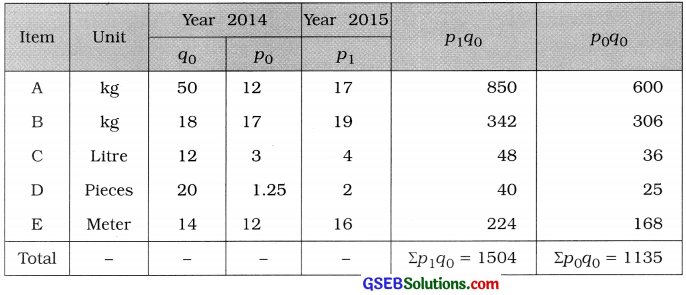Cost of living index number by total expenditure method:
I = $$\frac{\Sigma p_{1} q_{0}}{\Sigma p_{0} q_{0}}$$ × 100 = $$\frac{1504}{1135}$$ × 100 = 1.3251 × 100 = 132.51Question 4.
Compute the general index number for the production using the following data: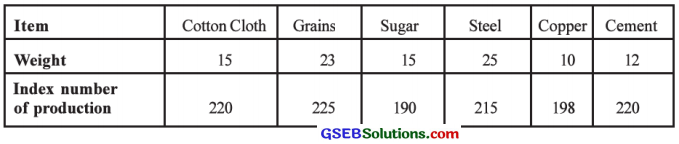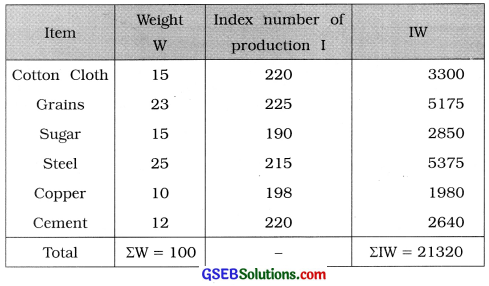General index number of production:
I = $$\frac{\Sigma \mathrm{IW}}{\Sigma \mathrm{W}}$$
= $$\frac{21320}{100}$$
= 213.20Question 5.
The details of expenditure on clothing for the worker class of a region are as follows. Find the index number for clothing by the total expenditure and family budget method.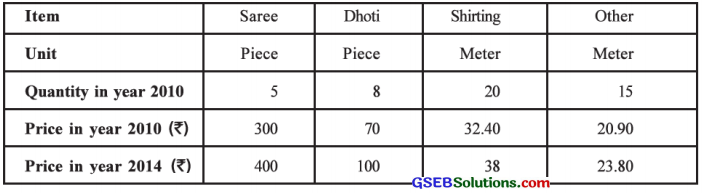Here, p0 = price in 2010, q0 = quantity in year 2010 and p1 = price in year 2014.

The table for calculation is prepared as follows: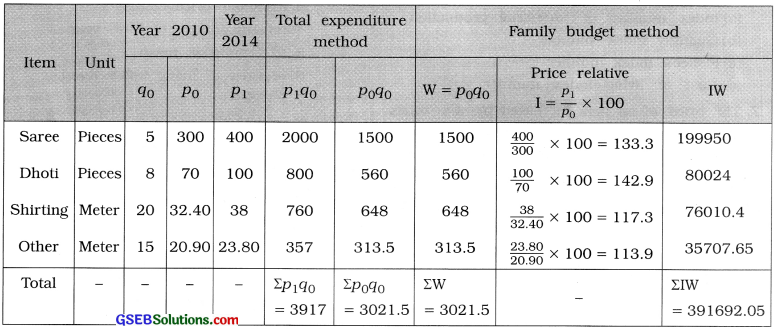Index number of clothing by total expenditure method:
I = $$\frac{\Sigma p_{1} q_{0}}{\Sigma p_{0} q_{0}}$$ × 100
= $$\frac{3917}{3021.5}$$ × 100
= 1.2964 × 100 = 129.64

Index number of clothing by family budget method:
I = $$\frac{\Sigma \mathrm{IW}}{\Sigma \mathrm{W}}$$
= $$\frac{391692.05}{3021.5}$$
= 129.64